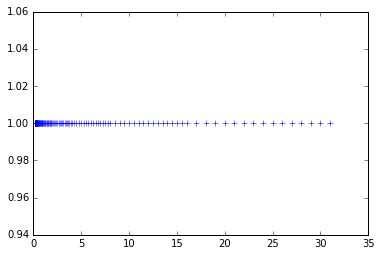# Density of Floating Point Numbers¶

This notebook enumerates all possible floating point nubmers in a floating point system and shows them in a plot to illustrate their density.

In :
import matplotlib.pyplot as pt
import numpy as np

In :
significand_bits = 4
exponent_min = -3
exponent_max = 4

fp_numbers = []
for exp in range(exponent_min, exponent_max+1):
for sbits in range(0, 2**significand_bits):
significand = 1 + sbits/2**significand_bits
fp_numbers.append(significand * 2**exp)

fp_numbers = np.array(fp_numbers)
print(fp_numbers)

pt.plot(fp_numbers, np.ones_like(fp_numbers), "+")
#pt.semilogx(fp_numbers, np.ones_like(fp_numbers), "+")


[  0.125       0.1328125   0.140625    0.1484375   0.15625     0.1640625
0.171875    0.1796875   0.1875      0.1953125   0.203125    0.2109375
0.21875     0.2265625   0.234375    0.2421875   0.25        0.265625
0.28125     0.296875    0.3125      0.328125    0.34375     0.359375
0.375       0.390625    0.40625     0.421875    0.4375      0.453125
0.46875     0.484375    0.5         0.53125     0.5625      0.59375
0.625       0.65625     0.6875      0.71875     0.75        0.78125
0.8125      0.84375     0.875       0.90625     0.9375      0.96875     1.
1.0625      1.125       1.1875      1.25        1.3125      1.375
1.4375      1.5         1.5625      1.625       1.6875      1.75
1.8125      1.875       1.9375      2.          2.125       2.25        2.375
2.5         2.625       2.75        2.875       3.          3.125       3.25
3.375       3.5         3.625       3.75        3.875       4.          4.25
4.5         4.75        5.          5.25        5.5         5.75        6.
6.25        6.5         6.75        7.          7.25        7.5         7.75
8.          8.5         9.          9.5        10.         10.5        11.
11.5        12.         12.5        13.         13.5        14.         14.5
15.         15.5        16.         17.         18.         19.         20.
21.         22.         23.         24.         25.         26.         27.
28.         29.         30.         31.       ]

Out:
[<matplotlib.lines.Line2D at 0x7f5020b580b8>]In [ ]: